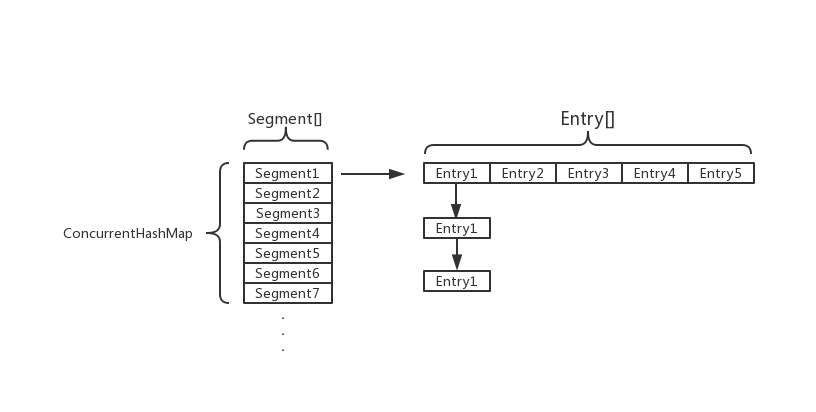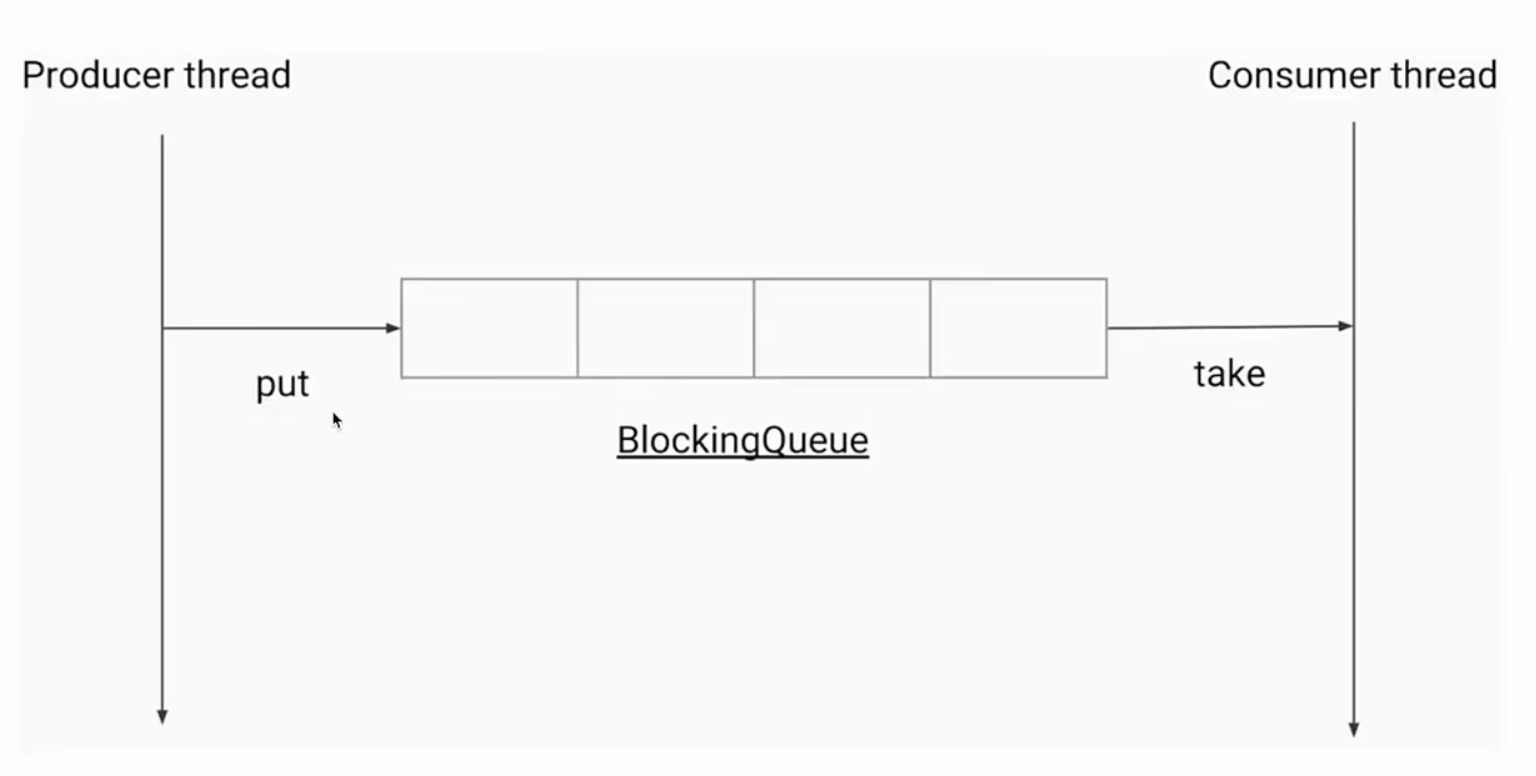# 一、ConcurrentHashMap源码分析

## 1.1 为什么要用ConcurrentHashMap

### 1.1.1 并发条件下使用HashMap

HashMap在并发条件执行put操作会引起死循环，因为多线程会导致HashMapEntry链表形成环状结构，也就是常见的链表成环，一旦形成环状的数据结构，Entrynext节点永远都不会为空，就会产生死循环获取Entry

### 1.1.2 并发条件下使用HashTable

HashTable容器使用Synchronized保证多线程安全，但在线程竞争激烈的情况下HashTable的效率十分低下，是因为当一个线程访问HashTable的同步方法时，其他线程也会访问HashTable的同步方法，会进入阻塞或轮询状态

## 1.2 ConcurrentHashMap结构

### JDK1.7JDK1.7中，ConcurrentHashMap是由Segment数组和Entry数组组成，Segment是一种可重入锁，在源码中直接继承的ReentrantLock

static class Segment<K,V> extends ReentrantLock implements Serializable {
private static final long serialVersionUID = 2249069246763182397L;
Segment(float lf) { this.loadFactor = lf; }
}

### JDK1.8

JDK1.8的实现已经抛弃了Segment分段锁机制，利用CAS+Synchronized来保证并发更新的安全，底层采用数组+链表+红黑树的存储结构

    public V put(K key, V value) {
return putVal(key, value, false);
}

final V putVal(K key, V value, boolean onlyIfAbsent) {
//ConcurrentHashMap的key不能为null
if (key == null || value == null) throw new NullPointerException();
//得到hash值
int hash = spread(key.hashCode());
//用于记录相应链表的长度
int binCount = 0;
for (Node<K,V>[] tab = table;;) {
Node<K,V> f; int n, i, fh;
//数组为空则初始化
if (tab == null || (n = tab.length) == 0)
tab = initTable();
//通过hash值找到对应的数组下标f，并且该数组中还没有元素
else if ((f = tabAt(tab, i = (n - 1) & hash)) == null) {
//使用CAS操作将新值放入table中
//          成功则退出循环
//          失败则表示有并发操作，进入下一次循环
if (casTabAt(tab, i, null,
new Node<K,V>(hash, key, value, null)))
break;                   // no lock when adding to empty bin
}
else if ((fh = f.hash) == MOVED)
//扩容导致数据迁移
tab = helpTransfer(tab, f);
//找到数组下标后，数组中已经有元素了，f是头结点
else {
V oldVal = null;
//获得头结点f的监视器锁
synchronized (f) {
if (tabAt(tab, i) == f) {
//头结点f的哈希值大于0，说明是链表
if (fh >= 0) {
//用于累加，记录链表长度
binCount = 1;
for (Node<K,V> e = f;; ++binCount) {
K ek;
//如果该节点的hash值等于待put的key的hash并且key值与节点key值“相等”则覆盖value
if (e.hash == hash &&
((ek = e.key) == key ||
(ek != null && key.equals(ek)))) {
oldVal = e.val;
if (!onlyIfAbsent)
e.val = value;
break;
}
Node<K,V> pred = e;
//如果到了链表末尾，则将新值插入链表最后
if ((e = e.next) == null) {
pred.next = new Node<K,V>(hash, key,
value, null);
break;
}
}
}
//红黑树
else if (f instanceof TreeBin) {
Node<K,V> p;
binCount = 2;
//调用红黑树的方法插入节点
if ((p = ((TreeBin<K,V>)f).putTreeVal(hash, key,
value)) != null) {
oldVal = p.val;
if (!onlyIfAbsent)
p.val = value;
}
}
}
}
if (binCount != 0) {
if (binCount >= TREEIFY_THRESHOLD)
//链表长度大于8转为红黑树
treeifyBin(tab, i);
if (oldVal != null)
return oldVal;
break;
}
}
}
return null;
}
    public V get(Object key) {
Node<K,V>[] tab; Node<K,V> e, p; int n, eh; K ek;
int h = spread(key.hashCode());
if ((tab = table) != null && (n = tab.length) > 0 &&
(e = tabAt(tab, (n - 1) & h)) != null) {
//判断头节点是否就是要查找的元素
if ((eh = e.hash) == h) {
if ((ek = e.key) == key || (ek != null && key.equals(ek)))
return e.val;
}
//hash值小于0，说明链表已经转为红黑树
else if (eh < 0)
return (p = e.find(h, key)) != null ? p.val : null;
//遍历链表获得value
while ((e = e.next) != null) {
if (e.hash == h &&
((ek = e.key) == key || (ek != null && key.equals(ek))))
return e.val;
}
}
return null;
}


# 二、CopyOnWriteArrayList分析

## 实现原理及源码分析

CopyOnWriteArrayList在写操作情况下是通过拷贝并写入的操作完成写操作，也就是在操作一条数据的时候会先拷贝一个新的集合，将原有的数据拷贝到新集合中进行操作，最后将新集合覆盖旧集合

public boolean add(E e) {
synchronized (lock) {
Object[] es = getArray();
int len = es.length;
es = Arrays.copyOf(es, len + 1);
es[len] = e;
setArray(es);
return true;
}
}

        /**
* Not supported. Always throws UnsupportedOperationException.
* @throws UnsupportedOperationException always; {@code remove}
*         is not supported by this iterator.
*/
public void remove() {
throw new UnsupportedOperationException();
}

private transient volatile Object[] array;

final Object[] getArray() {
return array;
}

public E get(int index) {
return elementAt(getArray(), index);
}

static <E> E elementAt(Object[] a, int index) {
return (E) a[index];
}

## 缺点

• 只保证数据的最终一致性，不保证实时一致性
• 因为CopyOnWriteArrayList的写主要是通过复制拷贝完成，所以在操作大批量的数据情况下，内存消耗代价很大

## 三、阻塞队列BlockingQueue1. 支持阻塞的插入方法：意思是当队列满时，队列会阻塞插入元素的线程，直到队列不满
2. 支持阻塞的移除方法：意思是在队列为空时，获取元素的线程会等待队列变为非空

• 抛出异常：当队列满时，如果再往队列里插入元素，会抛出IIegalStateException(“Queue
full”)异常。当队列为空时，从队列里获取元素会抛出NoSuchElementException异常
• 返回特殊值：当往队里插入元素时，会返回是否插入成功，如果是移除方法，则取出元素为空时返回null
• 一直阻塞：当阻塞队列满时，如果生产者线程往队列put元素，队列会一直阻塞生产者线程，直至队列不满或线程中断退出。当队列为空，如果消费者从队列里take时，队列会阻塞消费者线程，直至队列不为空
• 超时退出：超过阻塞队列指定的响应时间，生产者或消费者线程就会退出

### JUC包下的阻塞队列

• ArrayBlockQueue：数组组成的有界阻塞队列
• LinkedBlockingQueue：链表组成的无界阻塞队列
• PriorityBlockingQueue：支持优先级排序的无界阻塞队列
• DelayQueue：使用优先级队列实现的无界阻塞队列
• SynchronousQueue：不存储元素的阻塞队列
• LinkedTransferQueue：链表组成的无界阻塞队列
• LinkedBlockingDeque：链表组成的双向阻塞队列